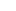Please enable JavaScript to view this page content properly Log In | Sign Up
 EMA Envelope SMA Envelope Percent Channels Darvas Box Donchian Channels Keltner Channels Bollinger Bands
 EMA Envelope Action Price Crossed Above Upper Bound of EMA Envelope(50,15)Price Crossed Above Lower Bound of EMA Envelope(50,15)Price Crossed Below Upper Bound of EMA Envelope(50,15)Price Crossed Below Lower Bound of EMA Envelope(50,15)Price Touched Above Upper Bound of EMA Envelope(50,15)Price Touched Above Lower Bound of EMA Envelope(50,15)Price Touched Below Upper Bound of EMA Envelope(50,15)Price Touched Below Lower Bound of EMA Envelope(50,15)Price Bounced Up From Upper Bound of EMA Envelope(50,15)Price Bounced Up From Lower Bound of EMA Envelope(50,15)Price Bounced Down From Upper Bound of EMA Envelope(50,15)Price Bounced Down From Lower Bound of EMA Envelope(50,15)Price Is Above Upper Bound of EMA Envelope(50,15)Price Is Above Lower Bound of EMA Envelope(50,15)Price Is Below Upper Bound of EMA Envelope(50,15)Price Is Below Lower Bound of EMA Envelope(50,15)Envelopes (Trading Bands) - Technical Analysis from A to Z An envelope is comprised of two moving averages. One moving average is shifted upward, and the second moving average is shifted downward. Envelopes define the upper and lower boundaries of a security's normal trading range. A sell signal is generated when the security reaches the upper band, whereas a buy signal is generated at the lower band. The optimum percentage shift depends on the volatility of the security - the more volatile, the larger the percentage.Bollinger Bands - Technical Analysis from A to Z Bollinger Bands are similar to moving average envelopes. The basic interpretation of Bollinger Bands is that prices tend to stay within the upper- and lower-band. The distinctive characteristic of Bollinger Bands is that the spacing between the bands varies based on the volatility of the prices. During periods of extreme price changes (i.e., high volatility), the bands widen to become more forgiving. During periods of stagnant pricing (i.e., low volatility), the bands narrow to contain prices.